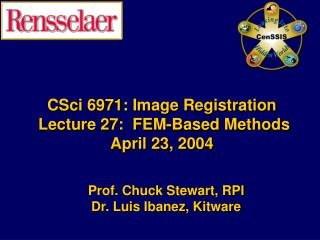DownloadDownload PresentationCSci 6971: Image Registration Lecture 27: FEM-Based Methods April 23, 2004

# CSci 6971: Image Registration Lecture 27: FEM-Based Methods April 23, 2004

Download Presentation## CSci 6971: Image Registration Lecture 27: FEM-Based Methods April 23, 2004

- - - - - - - - - - - - - - - - - - - - - - - - - - - E N D - - - - - - - - - - - - - - - - - - - - - - - - - - -
##### Presentation Transcript

1. CSci 6971: Image Registration Lecture 27: FEM-Based MethodsApril 23, 2004 Prof. Chuck Stewart, RPI Dr. Luis Ibanez, Kitware

2. Open Source Disclaimer Many of the slides in this talk were taken from the ITK course presented at MICCAI 2003 by Dr. James Gee (U. Penn)Brian Avants (U. Penn)Tessa Sundaram (U. Penn)Dr. Lydia Ng (Insightful Corp.) Of course, any errors… are mine. Lecture 27

3. Deformable Registration Finite Element Methodsfor Deformable Registration Lecture 27

4. Deformable Transformation y y Transform x x Fixed Image Moving Image Lecture 27

5. Deformable Transformation y y Transform x x Fixed Image Moving Image Lecture 27

6. Deformable Transformation y x Lecture 27

7. Deformable Transformation y x Lecture 27

8. FEM Grid y FEM Grid Resampling Grid x Lecture 27

9. FEM Grid y FEM Grid x Lecture 27

10. FEM Grid y FEM Grid Computed Deformation x Lecture 27

11. FEM Grid y FEM Grid Displacements Forces x Lecture 27

12. FEM Iterative Linear System Finite Element Methods F Vector N Forces U Vector N Displacements K Matrix NxN Regularization Lecture 27

13. FEM Iterative Linear System Finite Element Methods F K U F U = ● K Lecture 27

14. FEM Iterative Linear System N = Number of Nodes N x N N N = ● U F K Lecture 27

15. FEM Iterative Linear System Iteratively Solving a Linear System K U F = ● Linearization of a Physical Model Image based forces Node Displacements Lecture 27

16. FEM Energy Formulation Find registration transformation that maximizes Lecture 27

17. FEM Energy Formulation Benefits • Intuitive; easier to express constraints • Powerful numerical solutions available • Optimality of solutions; easier to debug Limitations • Difficult / overhead to implement Lecture 27

18. Deformable Registration To solve the deformation, consider only displacements of the form Lecture 27

19. Deformable Registration Linear Elements Lecture 27

20. Deformable Registration α1 Element φ1 Shape Function Lecture 27

21. Deformable Registration Element φ2 α2 Shape Function Lecture 27

22. Deformable Registration α3 φ3 Element Shape Function Lecture 27

23. Deformable Registration u α3 φ3 Element α1 φ1 φ2 α2 Shape Functions Lecture 27

24. Deformable Registration Higher Order Elements Lecture 27

25. Deformable Registration α1 Element φ1 Shape Function Lecture 27

26. Deformable Registration α4 φ4 Element Shape Function Lecture 27

27. Deformable Registration Element φ2 α2 Shape Function Lecture 27

28. Deformable Registration φ5 α5 Element Shape Function Lecture 27

29. Deformable Registration α3 φ3 Element Shape Function Lecture 27

30. Deformable Registration φ6 α6 Element Shape Function Lecture 27

31. Deformable Registration α4 φ4 u α3 φ3 Element φ6 α1 α6 φ1 φ5 α5 φ2 α2 Shape Functions Lecture 27

32. Deformable Registration Substitute uhinto E, then minimizing with respect to αi: Lecture 27

33. BSplines Grid & Image Grid Calculation are made in an Element by Element basis Lecture 27

34. BSplines Grid & Image Grid Elements are connected at Nodes at which the displacement is solved Lecture 27

35. BSplines Grid & Image Grid Efficiency is gained by elemental computation Lecture 27

36. BSplines Grid & Image Grid Domain subdivision (Mesh) can be tailored to the underlying geometry of the image. Lecture 27

37. FEM Solver Start Iteration Loop • Begin Loop by making physical assumptions and then taking the derivative of the similarity metric. • End loop when the solution stabilizes. Physical Assumptions New Solution Solve Image Metric Derivative End Iteration Loop Lecture 27

38. FEM Solver Start Iteration Loop K Physical Assumptions Solve New Solution U Image Metric Derivative F End Iteration Loop Lecture 27

39. K U F = ● FEM Solver Start Iteration Loop K U Unew F If ( Unew – Uold) < ε then Stop Lecture 27

40. KU=F in Code itk::FEMRegistrationFilter::IterativeSolve() itk::FEMSolver::AssembleK() FEMSolver :: AddSolution() FEMSolver:: Solve() FEMSolver::AssembleF() calls FEMImageMetricLoad::Fe() Lecture 27

41. FEM-Based Registration Options Element Type • Triangles • Quadrilaterals • Hexahedra • Tetrahedra Lecture 27

42. FEM-Based Registration Options Continuum / Physical Model • Linear elasticity • Membrane • Other specialized Lecture 27

43. FEM-Based Registration Options Mesh geometry • Uniform grid vs. adaptive • Anatomy-specific mesh Lecture 27

44. FEM-Based Registration Options Metric • Mean square • Normalized cross-correlation • Mutual information • Pattern intensity Lecture 27

45. ITK FEM Library Introduction to the ITK Finite Element Library Lecture 27

46. ITK FEM Library Library for solving general FEM problems • Object oriented • C++ classes are used to • specify the geometry and behavior of the elements • apply external forces and boundary conditions • solve problem and post-process the results Lecture 27

47. ITK FEM Library Applications • Mechanical modeling • Image registration Lecture 27

48. FEM Basics • Mesh • Nodes • Points in space where solutions are obtained • Elements • e.g., 2-D triangular elements • Loads • e.g., gravity (body) load • Boundary conditions • e.g., nodes fixed in space Lecture 27

49. ITK FEM Elements • Core of the library is the Element class • Code is in two functionally independent parts • Geometry and Physics • Arbitrarily combined to create new elements • Problem domain is specified by a mesh Geometry Physics Lecture 27

50. Loads Classes that apply external forces (loads) to elements • Various types • Easily extensible Lecture 27